Search IntMath
Close

450+ Math Lessons written by Math Professors and Teachers

5 Million+ Students Helped Each Year

1200+ Articles Written by Math Educators and Enthusiasts

Simplifying and Teaching Math for Over 23 Years

# IntMath Newsletter: Graph mystery, science GIFs

By Murray Bourne, 29 Mar 2017

29 Mar 2017

1. What's the correct graph of sec(arccos(x))?
2. Resource: Science GIFs
3. Math in the news: income inequality
4. Math movie: Benjamin Banneker
5. Math puzzles
6. Final thought: Priorities

## 1. What's the correct graph of sec(arccos(x))?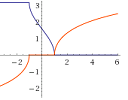Here we solve another graph mystery - what is the correct domain for y = sec(arccos(x))? And why do different software packages show similar, but different graphs? What's the correct graph of sec(arccos(x))?

## 2. Resource: Science GIFsHere's an interesting collection of animated GIFs showing a range of science concepts. I especially liked "Formation of a Nor'easter" and "Wingtip Vortices". See: Science gifs

## 3. Math in the news - curbing income inequality

There's been discussion in the UK about imposing a maximum wage on top executives as a multiple of the wage of the lowest paid employee. According to Pay caps are neither economically crazy nor morally wrong in the Irish Times:

In the private sector in the US the average chief executive is paid more than 500 times the pay of the average blue-collar worker.

Some countries are considering a Universal Basic Income (e.g. Finland), while others agonize over whether to ensure workers get at least a basic minimum wage.

So what wage are we really worth? One commonly used model of wages as a function of the amount of education and experience is the Mincer Earnings Function, expressed as: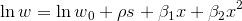where

w = earnings

w0 = earnings of someone with no education nor experiences

s = years of schooling

x = years of potential labor market experience

ρ is the return due to schooling

β1 and β2 are the returns due to experience

So next time you go to your boss to ask for a raise, take this equation with you.

## 4. Math movie: The exceptional life of Benjamin BannekerSelf-taught mathematician and astronomer Benjamin Banneker excelled despite little formal education and prejudice. Math movie: The exceptional life of Benjamin Banneker

## 5. Math puzzles

The puzzle in the last IntMath Newsletter asked about the dimensions and volumes of 2 boxes made from a square sheet of metal.

To make a square open box, we need to cut smaller (green) squares of side s, like this: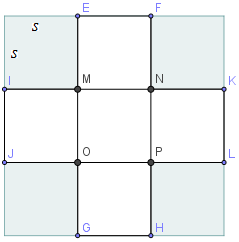In terms of v, the dimensions of the original square are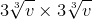In terms of s, the dimensions of the original square are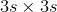Some of your answers started with a square of side x and found its side length to be: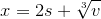or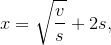both of which of course are the same as x = 3s.

Correct answers for this part were given by Jatin, Saikrishna, Jeel, Don, Don A, and Chris.

For the bonus part, we need to maximise the length of each of the cube sides, while having enough material left over for the top.

### Solution 1

Here's one way to do it, where we cut out the 4 green triangles. The smaller triangular tips are then folded in to create the top.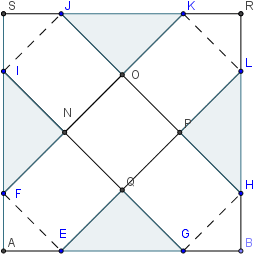To find the size of those triangles that need to be cut out, and the final volume, we proceed as follows.

Let the final cube dimensions be m × m × m.

We find distance JK by observing OJK is a right isosceles triangle with equl sides m. Using Pythagoras, we find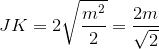We also have (so the triangle tips fold in and fit properly)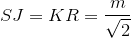So the total length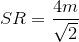But we know that length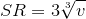So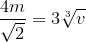This gives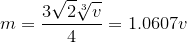So the volume of our maximized cube is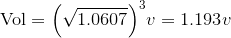That is, it's 1.193 times the volume of our open box.

Saikrishna suggested the above solution.

But does that give us the maximum volume? There's a lot of unused metal left over (the green triangle parts).

### Solution 2 - use all the metal

The following approach (suggested by some of you) uses all of the metal sheet. We know we need 6 squares to make the closed box, and thosse squares will have 1/6 the area of the metal sheet.

So if we let the original sheet be 3 × 3 = 9 cm2, (for convenience), then each of the 6 squares making the box has equal area 9/6 = 1.5 cm2.

This means the side of each square is √1.5 = 1.224745.

So the volume of the box is given by: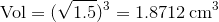That is, our closed box using all the metal will have volume 1.87 times the volume of the first open box.

Here's how we would need to cut the sheet. The 4 squares shown have dimensions √1.5 × √1.5.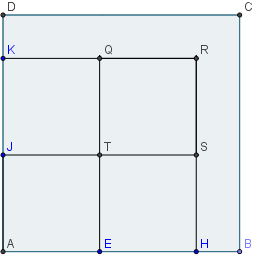Next, I've cut out section above KQ and placed it above rectangle QRIG. You can see it's not quite big enough (the height is 1.1 cm, not 1.2247.)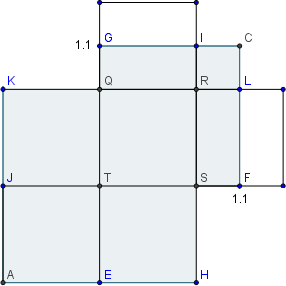Similarly, I cut out the rectangle to the right of HS and placed it to the right of SFLR. It's also not quite big enough.

So we would need to cut strips off the remaining square RLCI to make actual squares. This would be quite troublesome, since there would be several little strips to weld together.

While Solution 2 does maximise the volume, from a manufacturing point of view, the most likely approach adopted by a factory would be to use slightly smaller squares.

### Solution 3 - use most of the metal

If we use sides of dimension 1.2 cm, it works nicely, with minimal cutting involved and yielding a volume of 1.728 cm3) and only a small amount of left over metal.

The best mathematical solution is not always the most practical answer.

### New math puzzle: Integers

For integers m and n, how many solutions (m, n) are there such that m2 + n2 ≤ 12?

You can leave your responses here.

## 6. Final thought: Priorities

There is a flurry of excitement regarding Elon Musk's plans to send people to Mars.

But I don't get it.

Don't get me wrong - I'm totally in favor of scientific exploration, but I can't help but think it would be far wiser to spend the considerable sums involved on fixing more pressing problems here on Earth, like climate change, improving educational opportunities, ramping up the water supply and improving its quality, and reducing air pollution, poverty and income inequality.[Image source]

Now that would leave a more meaningful and lasting legacy, wouldn't it?

Until next time, enjoy whatever you learn.

1. Don A says:

I found 12 positive integer combinations using trial and error: 0,0; 0,1; 1,0; 0,2; 2,0; 0,3; 3,0; 1,2; 2,1; 1,3 and 3,1
Using the negative integers adds another 18 combinations for a total of 30.

2. Matlunix says:

{(0,0), (0,1), (0,2), (0,3), (1,0), (1,1), (1,2), (1,3), (2,0), (2,1), (2,2), (3,0), (3,1), (-3,-1), (-3,0), (-2,-2), (-2,-1), (-2,0), (-1,-3), (-1,-2), (-1,-1), (-1,0), (0,-3), (0,-2), (0,-1), (0,0)}

Psuedocode to acheive this task. Hopefully this is correct.
1. Begin loop from i = 0 to 9: #loop_1
2. Begin loop from j = 0 to 9: #loop_2
3. Branch: if i²+j² <= 12: #branch_1
4. Print message "%s,%s"%(i,j)
5. End #branch_1
6. End #loop_2
7. End #loop_1

1. Begin loop from i = -10 to 0: #loop_1
2. Begin loop from j = -10 to 0: #loop_2
3. Branch: if i²+j² <= 12: #branch_1
4. Print message "%s,%s"%(i,j)
5. End #branch_1
6. End #loop_2
7. End #loop_1

3. Don A says:

Your priorities are far different from mine. I would not spend a cent to reduce global warming. We cannot even agree it is a problem, and the only solutions being offered are to basically go back to the days before man learned to control and use fire or reduce the population by orders of magnitude. Neither sounds like anything I would want to be a part of.

Like wise I do not see income inequality as a problem at all. The societies that have contributed the most wealth and there by improving the lives of the most people are those that rewarded productive work with economic advantage. You create wealth and therefore reduce poverty by rewarding those who work to improve things and motivate the less productive by increasing income inequality. Importantly you must assure the contributions being rewarded are the ones most beneficial to the happiness of others. That requires a free market to set prices. It does not require large expenditures of public funds. Availability and cost of clean water and air will take care of them selves if the market is allowed to work for all the people.

Maybe we need to keep the subject of this forum to math.

4. cccccttttt says:

Re: what is the real value of an hour of labor

A major social issue as an employer must now judge the cost effectiveness of supplanting certain human jobs with robots.

"The world of the future will be an ever more demanding struggle against the limitations of our intelligence," mathematician Norbert Wiener wrote in 1964, "not a comfortable hammock in which we can lie down to be waited upon by our robot slaves."

ct

5. Roger Evans says:

On final thought: priorities
Perhaps Murray the people doing this don't think we can ever solve the problems on Earth and we need to get some breeding humans away from this planet to try to ensure survival of the human race. Preferably they should be Americans?
Or maybe they think the Sun will start changing into a red giant much earlier than expected!
Kind regards,
Roger

6. jeel says:

For m^2+n^2<_12
Let m^2=a and n^2 =b where a and b are perfect squares
such numbers less than 12 are 0,1,4,9. So m or n can be 0,1,2,3,-1,-2,-3
using permutation and combination there are 49 possible combinations but m,n such as 3,3. 3,-3 2,3 2,-3 etc have to be ignored as they do not satisfy the condition total such 12 combinations have to be removed so the answer for m,n is 49-12=37

7. Sachin Munshi says:

For the math puzzle, to find the pairs of integers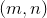such that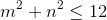, we proceed as follows. For the cases where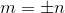or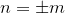, we have pairs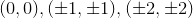accounting for a total of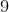integer pairs that satisfy the given inequality. For other cases where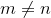, we have pairs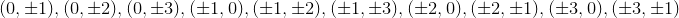accounting for a total of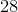additional integer pairs that satisfy the given inequality. Therefore, the grand total is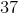pairs of integerssuch that.

8. DON MILLER says:

for m=0 to 3
for n=0 to 3
T= m^2 +n^2
if T<= 12 then
count =count + 1
endif
next
next

print count

COUNT = 13

9. paul holland says:

It's a worthy thought that we spend money on fixing Earth-bound problems rather than going to space. I don't quite agree.
There were ample problems in Europe in old times but the likes of Vasco Da Gama, Columbus went forth on far riskier trips than space travel now entails. What use were Einstein's theories when he first mooted them? Why have any new children when so many are starving?
Money spent on space is nothing compared to that spent on armaments, bonuses for bankers, salaries for politicians and weekend drunken binges.
We need something to aspire to, otherwise we just stagnate. Isn't that what happened to the Roman Empire?

10. Don A says:

11. Don A says:

"supplanting certain human jobs"
There are no "human jobs". Just jobs that we have not found a way to economically automate, YET.

12. Murray says:

@Don A: Why not good? This is how it has worked for all the math puzzles for some years. Responses are on the same page as the question, and published once the next Newsletter goes out.

13. Gerard Grufferty says:

Write the possibilities (m, n) in a "group" table:
m on the left and n at the top.

m\n -3 -2 -1 0 1 2 3

-3 (-3, -1) (-3, 0) (-3, 1)

-2 (-2, -2) (-2, -1) (-2, 0) (-2, 1) (-2, 2)

-1 (-1, -3) (-1, -2) (-1, -1) (-1, 0) (-1, 1) (-1,2) (-1, 3)

0 (0, -3) (0, -2) (0, -1) (0, 0) (0, 1) (0,2) (0, 3)

1 (1, -3) (1, -2) (1, -1) (1, 0) (1, 1) (1,2) (1, 3)

2 (2, -2) (2, -1) (2, 0) (2, 1) (2, 2)

3 (3, -1) (3, 0) (3, 1)

Counting: 3 + 5 + 7 + 7 + 7 + 5 + 3 = 37

14. Colin Morrison says:

Since 0,1,-1,2,-2,3,-3 are all integers with 3sq plus 1sq being the largest combinations less than or equal to 12 there are 37 solutions to msq + nsq less than or equal to 12.

(0,0)= 1

(1,0), (0+1), (-1,0) ,(0,-1),similarly for (2,0)and(3,0);total=12

(1,1), (1,-1),(-1,1), (-1,-1) = 4, similarly for (1,2, (2,1), (2,2)(1,3), (3,1): total = 24

total (m,n) solutions = 37

15. Bawari Lakanwal says:

If we put m=x and n=y then x^2+y^2=12 is a circle with the center (0,0) and radius of sqrt (12) units. Into this circle there are 28 pairs of integers(m,n), each pair has two different integers and indicates an inner point into the circle. Here they are: (0,1), (1,0), (0,-1), (-1,0), (0,2), (2,0), (0,-2), (-2, 0), (0,3), (3,0), (0,-3), (-3, 0), (1, 2), (2, 1),(-1, -2), (-2, -1), (1, -2), (-2, 1), (-1, 2), (2, -1), (1, 3), (3, 1), (-1, -3), (-3, -1), (1,-3), (-3, 1), (-1, 3), (3, -1).

16. Thomas A Buckley says:

There are 24 combinations.
Six all positive integers for (m,n)
(1,1), (2,1), (3,1), (1,2), (2,2), (1,3)
Same six combos again, but all m's negative, ie (-1,1) for 1st
Initial same six combos again, but all n's negative, ie (1,-1) for 1st
Initial same six combos again, but all m's & n's negative, ie (-1,-1) for 1st

So total is 6 x 4 = 24 = Answer

17. ernest says:

37

18. Stephen says:

@Dan A: I feel sorry for you, since it's known that selfish people are generally less happy. You really should get out of your office and go see the world, where the free market you think is so wonderful has not provided benefits to all. Your view - and opportunities - from an obviously privileged position is not one that can ever be shared by those born into abject poverty.

Last time I looked, photovoltaics and battery storage is hardly going "back to the days before man learned to control and use fire".

Scientists do agree about global warming - it's such a shame the clueless politicians do not. Go visit Kiribati for a first-hand view.

Murray has every right to express his concerns about the world. Math is not just about algebra.

### Comment Preview

HTML: You can use simple tags like <b>, <a href="...">, etc.

To enter math, you can can either:

1. Use simple calculator-like input in the following format (surround your math in backticks, or qq on tablet or phone):
a^2 = sqrt(b^2 + c^2)
(See more on ASCIIMath syntax); or
2. Use simple LaTeX in the following format. Surround your math with $$ and $$.
$$\int g dx = \sqrt{\frac{a}{b}}$$
(This is standard simple LaTeX.)

NOTE: You can mix both types of math entry in your comment.